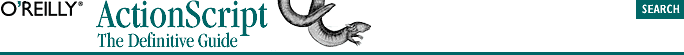home | O'Reilly's CD bookshelfs | FreeBSD | Linux | Cisco | Cisco Exam## 2.2. Assigning Variables

`variableName = value;`

where variableName is the name of a variable, and value is the data we're assigning to that variable. Here's an applied example:

`bookTitle = "ActionScript: The Definitive Guide";`

On the left side of the equal sign, the word bookTitle is the variable's name (its identifier). On the right side of the equal sign, the phrase "ActionScript: The Definitive Guide" is the variable's value -- the datum you're depositing. The equal sign itself is called the assignment operator. It tells Flash that you want to assign (i.e., deposit) whatever is on the right of the equal sign to the variable shown on the left. If the variable on the left doesn't exist yet, Flash creates it (though relying on the interpreter to implicitly create variables isn't recommended).

Here are two more variable assignment examples:

```speed = 25;
output = "thank you";```

The first example assigns the integer 25 to the variable speed, showing that variables can contain numbers as well as text. We'll see shortly that they can contain other kinds of data as well. The second example assigns the text "thank you" to the variable output. Notice that we use straight double quotation marks (" ") to delimit a text string in ActionScript.

Now let's look at a slightly more complicated example that assigns y the value of the expression 1 + 5:

`y = 1 + 5;`

When the statement y = 1 + 5; is executed, 1 is first added to 5, yielding 6, and then 6 is assigned to y. The expression on the right side of the equal sign is evaluated (calculated or resolved) before setting the variable on the left side equal to that result. Here we assign an expression that contains the variable y to another variable, z:

`z = y + 4;`

Once again, the expression on the right of the equal sign is evaluated and the result is then assigned to z. The interpreter retrieves the current value of y (it checks its account balance, so to speak) and adds 4 to it. Because the value of y is 6, z will be set to 10.

The syntax to assign any data -- whether numbers, text, or any other type -- to a variable is similar regardless of the datatype. For example, we haven't studied arrays yet, but you should already recognize the following as a variable assignment statement:

`myList = ["John", "Joyce", "Sharon", "Rick", "Megan"];`

As before, we put the variable name on the left, the assignment operator (the equal sign) in the middle, and our desired value on the right.

To assign the same value to multiple variables in a hurry, we may piggyback assignments alongside one another, like this:

`x = y = z = 10;`

Variable assignment always works from right to left. The preceding statement assigns 10 to z, then assigns the value of z to y, then assigns the value of y to x.